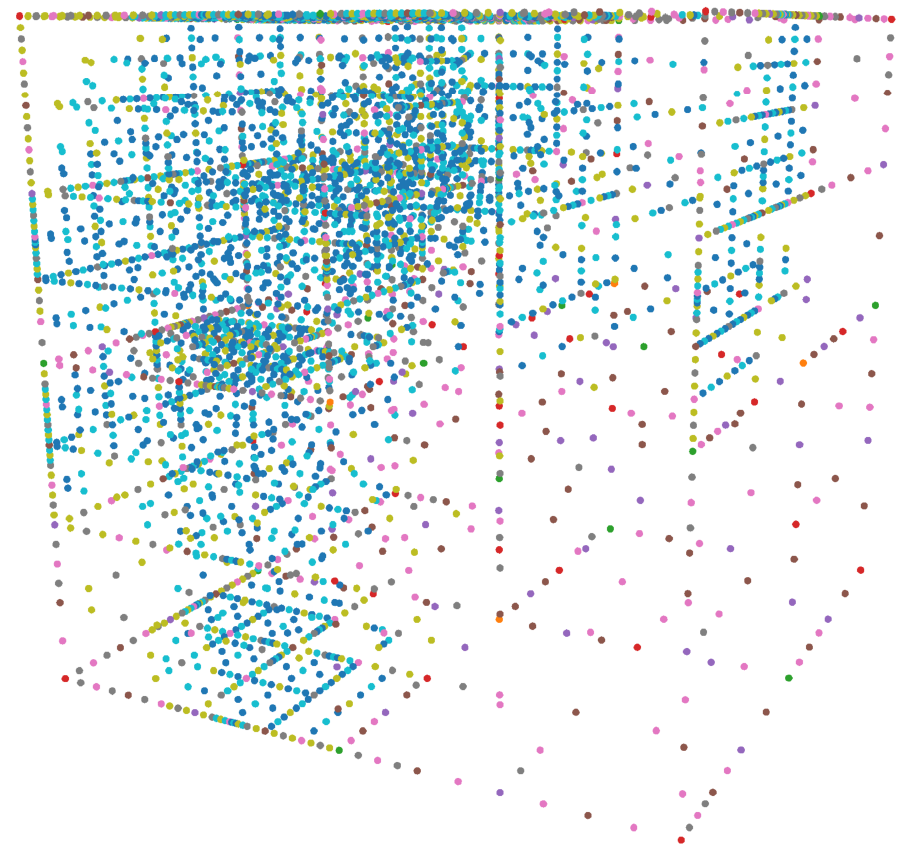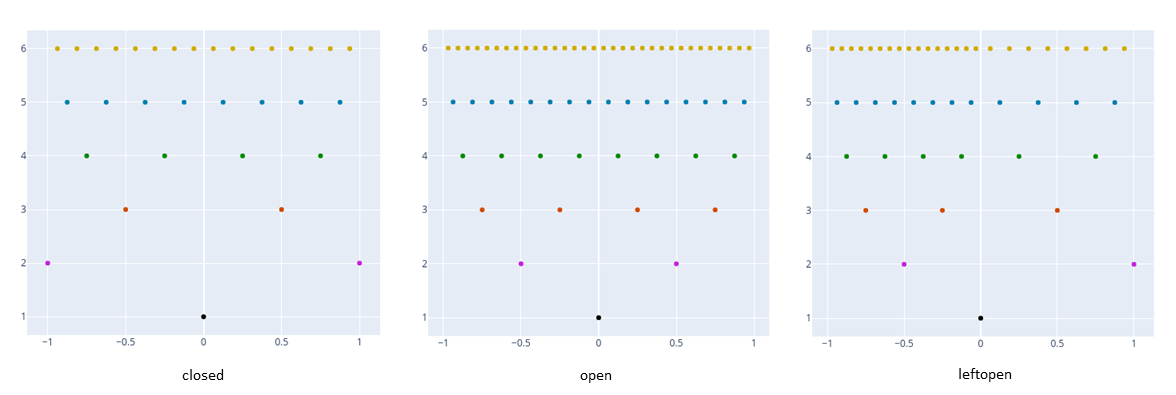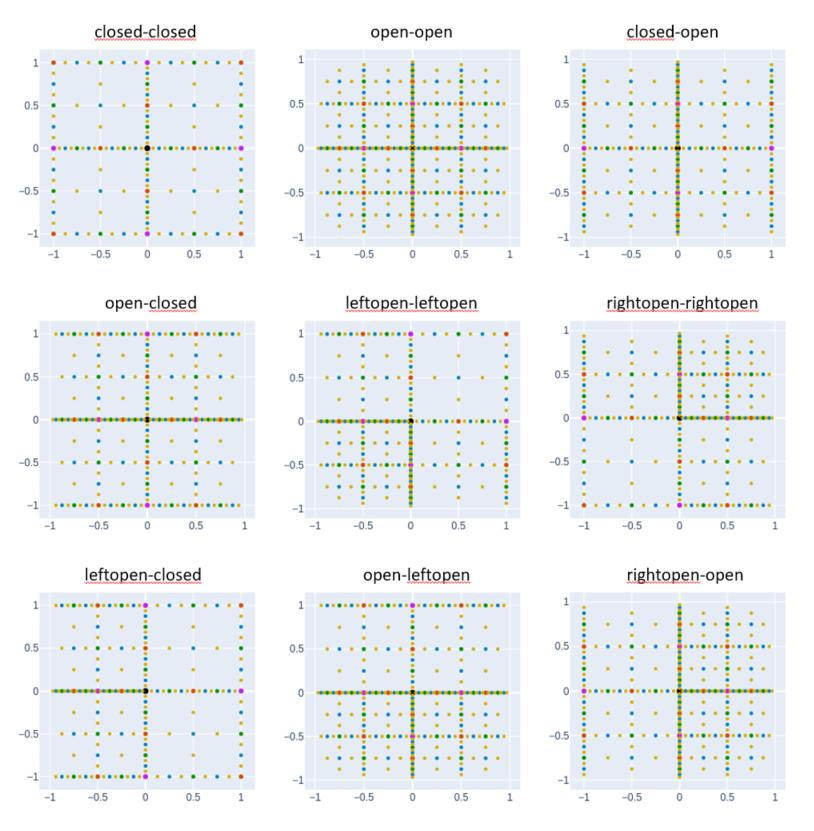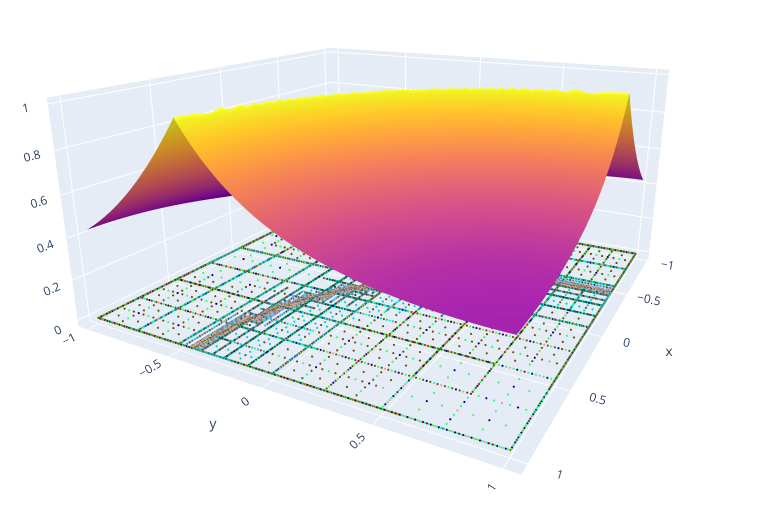## DistributedSparseGrids.jl

An hierarchical adaptive sparse-grid implementation in the julia language
Author baxmittens
Popularity
15 Stars
Updated Last
7 Months Ago
Started In
February 2022# DistributedSparseGrids.jl

A Julia library implementing an Adaptive Sparse Grid collocation method for integrating memory-heavy objects generated on distributed workers.

For an alternative implementation, see AdaptiveSparseGrids.jl.

## Introduction

Efficient methods for the numerical integration (or interpolation) of one-dimensional functions can be directly applied to the multidimensional case via tensor-product constructions. However, the higher the number of dimensions, the less efficient this approach is. A problem which is also known as the curse of dimensionality.

Any integral

$$\int_{a_1}^{b_1}\cdot\cdot\cdot\int_{a_n}^{b_n} f(x_1,...,x_n) \mathrm{d}x_1\cdot\cdot\cdot\mathrm{d}x_n$$

can be mapped onto the hypercube $[-1,1]^n$ by means of coordinate transformation. Therefore, the problem of integrating multidimensional functions can be studied on the hypercube without loss of generality.

To mitigate the curse of dimensionality that occurs in the integration or interpolation of multidimensional functions on the hypercube, sparse grids use Smolyak's quadrature rule. This is particularly useful if the evaluation of the underlying function is costly. In this library, an Adaptive Sparse Grid Collocation method with a local hierarchical Lagrangian basis, first proposed by Ma and Zabaras (2010), is implemented. For more information about the construction of Sparse Grids, see e.g. Gates and Bittens (2015).

## Install

import Pkg
Pkg.add("DistributedSparseGrids")

## Implemented features

• Nested one-dimensional Clenshaw-Curtis rule
• Smolyak's sparse grid construction
• local hierarchical Lagrangian basis
• different pointsets (open, closed, halfopen)
• distributed function evaluation with Distributed.remotecall_fetch
• multi-threaded calculation of basis coefficients with Threads.@threads
• usage of arbitrary input, collocation point, and return types
• integration
• experimental: integration over $X_{\sim (i)}$ (the $X_{\sim (i)}$ notation indicates the set of all variables except $X_{i}$).

## Usage

### General remarks

• The quality of the error prediction depends on the number of collocation points. Therefore, for only a few collocation points, adaptive refinement may fail. Therefore it is recommended to generate some initial levels before using adaptive refinement (see examples).
• The interpolation is based on a local Lagrangian basis. Functions with discontinuities cannot be approximated.
• Sparse grid interpolation and integration will work best with a number of dimension between 1 and 6.
• The domain of the sparse grid is always $[-1,1]^n$. The user is responsible to map the input onto this domain.

### Point sets

When using sparse grids, one can choose whether the $2d$ second-level collocation points should lay on the boundary of the domain or in the middle between the origin and the boundary. (There are other choices as well.) This results in two different sparse grids, the former with almost all points on the boundary and on the coordinate axes, the latter with all points in the interior of the domain. Since one can choose for both one-dimensional children of the root point individually, there exist a multitude of different point sets for Sparse Grids.

using DistributedSparseGrids
using StaticArrays

function sparse_grid(N::Int,pointprops,nlevel=6,RT=Float64,CT=Float64)
# define collocation point
CPType = CollocationPoint{N,CT}
# define hierarchical collocation point
HCPType = HierarchicalCollocationPoint{N,CPType,RT}
# init grid
asg = init(AHSG{N,HCPType},pointprops)
#set of all collocation points
cpts = Set{HierarchicalCollocationPoint{N,CPType,RT}}(collect(asg))
# fully refine grid nlevel-1 times
for i = 1:nlevel-1
union!(cpts,generate_next_level!(asg))
end
return asg
end

# define point properties
#	1->closed point set
# 	2->open point set
#	3->left-open point set
#	4->right-open point set

asg01 = sparse_grid(1, @SVector )
asg02 = sparse_grid(1, @SVector )
asg03 = sparse_grid(1, @SVector )

asg04 = sparse_grid(2, @SVector [1,1])
asg05 = sparse_grid(2, @SVector [2,2])
asg06 = sparse_grid(2, @SVector [1,2])
asg07 = sparse_grid(2, @SVector [2,1])
asg08 = sparse_grid(2, @SVector [3,3])
asg09 = sparse_grid(2, @SVector [4,4])
asg10 = sparse_grid(2, @SVector [3,1])
asg11 = sparse_grid(2, @SVector [2,3])
asg12 = sparse_grid(2, @SVector [4,2])### Integration and Interpolation

asg = sparse_grid(4, @SVector [1,1,1,1])

#define function: input are the coordinates x::SVector{N,CT} and an unique id ID::String (e.g. "1_1_1_1")
fun1(x::SVector{N,CT},ID::String) = sum(x.^2)

# initialize weights
@time init_weights!(asg, fun1)

# integration
integrate(asg)

# interpolation
x = rand(4)*2.0 .- 1.0
val = interpolate(asg,x)	

### Distributed function evaluation

asg = sparse_grid(4, @SVector [1,1,1,1])

# add worker and register function to all workers
using Distributed
ar_worker = workers()
@everywhere begin
using StaticArrays
fun2(x::SVector{4,Float64},ID::String) = 1.0
end

# Evaluate the function on 2 workers
distributed_init_weights!(asg, fun2, ar_worker)

### Using custom return types

For custom return type T to work, following functions have to be implemented

import Base: +,-,*,/,^,zero,zeros,one,ones,copy,deepcopy

+(a::T, b::T)
+(a::T, b::Float64)
*(a::T, b::Float64)
-(a::T, b::Matrix{Float64})
-(a::T, b::Float64)
zero(a::T)
zeros(a::T)
one(a::T)
one(a::T)
copy(a::T)
deepcopy(a::T)

This is already the case for many data types. Below RT=Matrix{Float64} is used.

# sparse grid with 5 dimensions and levels
pointprops = @SVector [1,2,3,4,1]
asg = sparse_grid(5, pointprops, 6, Matrix{Float64})

# define function: input are the coordinates x::SVector{N,CT} and an unique id ID::String (e.g. "1_1_1_1_1_1_1_1_1_1"
# for the root poin in five dimensions)
fun3(x::SVector{N,CT},ID::String) = ones(100,100).*x

# initialize weights
@time init_weights!(asg, fun3)

### In-place operations

There are many mathematical operations executed which allocate memory while evaluting the hierarchical interpolator. Many of these allocations can be avoided by additionally implementing the inplace operations interface for data type T.

import LinearAlgebra, AltInplaceOpsInterface

LinearAlgebra.mul!(a::T, b::Float64)
LinearAlgebra.mul!(a:T, b::T, c::Float64)
AltInplaceOpsInterface.minus!(a::T, b::Float64)
AltInplaceOpsInterface.minus!(a::T, b::T)
AltInplaceOpsInterface.pow!(a::T, b::Float64)

For Matrix{Float64} this interface is already implemented.

# initialize weights
@time init_weights_inplace_ops!(asg, fun3)

### Distributed function evaluation and in-place operations

# initialize weights
@time distributed_init_weights_inplace_ops!(asg, fun3, ar_worker)

# Sparse Grid with 4 initial levels
pp = @SVector [1,1]
asg = sparse_grid(2, pp, 4)

# Function with curved singularity
fun1(x::SVector{2,Float64},ID::String) =  (1.0-exp(-1.0*(abs(2.0 - (x-1.0)^2.0 - (x-1.0)^2.0) +0.01)))/(abs(2-(x-1.0)^2.0-(x-1.0)^2.0)+0.01)

init_weights!(asg, fun1)

for i = 1:20
# call generate_next_level! with tol=1e-5 and maxlevels=20
cpts = generate_next_level!(asg, 1e-5, 20)
init_weights!(asg, collect(cpts), fun1)
end

# plot
import PlotlyJS
surfplot = PlotlyJS.surface(asg, 100)
gridplot = PlotlyJS.scatter3d(asg)
PlotlyJS.plot([surfplot, gridplot])### Plotting

#### 1d

# grid plots
PlotlyJS.scatter(sg::AbstractHierarchicalSparseGrid{1,HCP}, lvl_offset::Bool=false; kwargs...)
UnicodePlots.scatterplot(sg::AbstractHierarchicalSparseGrid{1,HCP}, lvl_offset::Bool=false)

# response function plots
UnicodePlots.lineplot(asg::AbstractHierarchicalSparseGrid{1,HCP}, npts = 1000, stoplevel::Int=numlevels(asg))
PlotlyJS.surface(asg::SG, npts = 1000, stoplevel::Int=numlevels(asg); kwargs...)

#### 2d

# grid plots
PlotlyJS.scatter(sg::AbstractHierarchicalSparseGrid{2,HCP}, lvl_offset::Float64=0.0, color_order::Bool=false)
UnicodePlots.scatterplot(sg::AbstractHierarchicalSparseGrid{2,HCP})
PlotlyJS.scatter3d(sg::AbstractHierarchicalSparseGrid{2,HCP}, color_order::Bool=false, maxp::Int=1)

# response function plot
PlotlyJS.surface(asg::AbstractHierarchicalSparseGrid{2,HCP}, npts = 20; kwargs...)

#### 3d

# grid plot
PlotlyJS.scatter3d(sg::AbstractHierarchicalSparseGrid{3,HCP}, color_order::Bool=false, maxp::Int=1)

## Next steps

• nonlinear basis functions
• wavelet basis

## Contributions, report bugs and support

Contributions to or questions about this project are welcome. Feel free to create a issue or a pull request on GitHub.

### Required Packages

View all packages

### Used By Packages

No packages found.